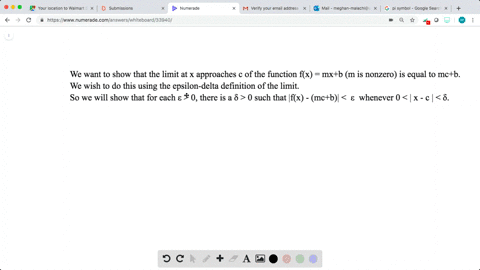Enroll in one of our FREE online STEM bootcamps. Join today and start acing your classes!View Bootcamps## Discussion

You must be signed in to discuss.

## Video Transcript

we were asked to prove the uniqueness of a limit. In other words, I suppose that for some function F, the LTD's Xa purchase see exists and is equal to l one. And we also have that a limited X purchase. CNF vexes equal toe l two. You want to show that l one is equal to l two using the formal definition of a limit. So first of all, let Epsilon be some positive real number. Then we know that by the definition of a limit, we confined Delta one greater than zero and adult it too greater than zero such that offering X one step saying For all I can write this says yes. If X lies between or X minus Sea lies between zero and Delta one, then we have that f of X minus. L one is less than epsilon. And if x minus c life between zero and Delta two, then it follows that absolute value of f of X minus. L two is less than epsilon. How are we going to combine these two statements? Well, let's pick Delta to be the minimum of Delta one in Delta two. Clearly since Delta one in Delta two are both great, and zero follows that Delta is greater than zero. And we have that if X minus sea lies between zero and Delta, then both of these statements above her. True. So we have that f of x minus. L one is less than up salon and f of X minus. L two is less than epsilon. So let's think about the difference between two possible limits l one and L two. So we have that the absolute value of l one minus l two. This is equal to f of X minus l two minus f of X minus l one and then by the triangle Inequality. This is going to be less stand or equal to absolute value of f of X minus l two plus the absolute value of f of X minus l one and we have from the previous sentence. Both of these quantities were going to be strictly less than epsilon. This is less than Absalon plus Epsilon, which is equal to two Absolute. So we have at this distance between l one and L two is one be less than 12 salon. But since epsilon is just some arbitrary positive number. And since l one and L two do not, and on Epsilon it follows that distance between l one. L two must be equal to zero because absolute eyes were greater than zero. Therefore, it follows. No one is equal to l two, which is what we wanted to prove. This shows that the limit, if it exists, is unique.

## Recommended Questions

#### You're viewing a similar answer. To request the exact answer, fill out the form below:

Our educator team will work on creating an answer for you in the next 6 hours.